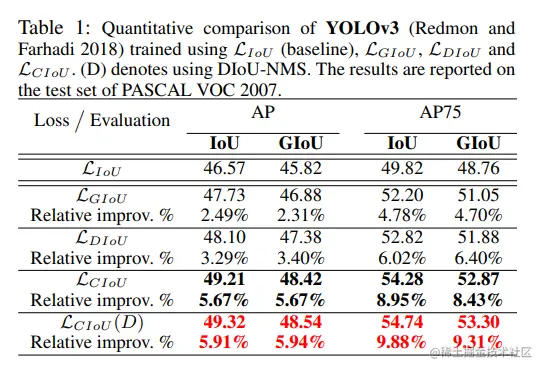# [target detection (8)] a thorough understanding of the loss function of the regression box of target detection -- the principles of IOU, giou, Diou and ciou and Python code

2022-02-02 06:12:26

Target detection includes two types of tasks , One is classification task , That is to classify the identified targets , The other is bbox Return mission , namely localization location , Loss regression is required for the predicted bounding box . This paper mainly expounds the design idea and related code implementation of regression box loss function in current mainstream target detection algorithms , Include L2 Loss、Smooth L1 Loss、IoU Loss、GIoU Loss、DIoU Loss and CIoU Loss.

## 1. Smooth L1 Loss

stay Faster RCNN in , The offset of the prediction bounding box offset Regression , Where the offset offset The definitions are as follows ：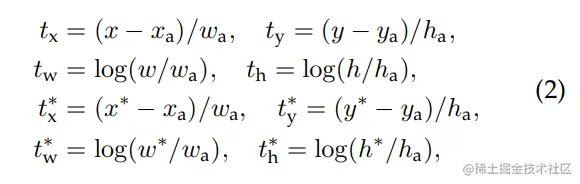Used in paper Smooth L1 Loss For the above offset Regression , The calculation method is as follows ：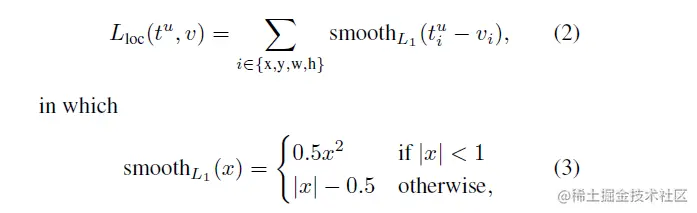Smooth L1 yes L1 and L2 The combination of , A combination of L1 and L2 The advantages of , Approaching 0 Within a certain range of L2 Loss, And outside this interval L1 Loss, You may have a deeper understanding by looking at the following images ：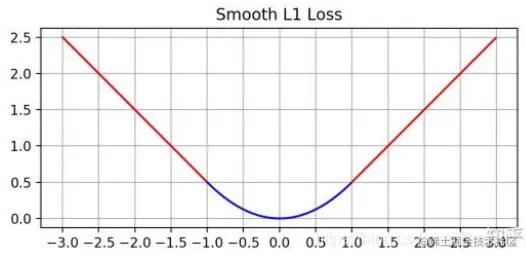Use Smooth L1 The benefits of ：

• L1 Loss The disadvantage is that 0 You can't lead , And lead to later training , Forecast value and ground truth The difference is very small ,L1  The absolute value of the derivative of the loss to the predicted value is still 1, and learning rate If it doesn't change , The loss function will fluctuate near the stable value , It is difficult to continue convergence to achieve higher accuracy .
• L2 Loss The disadvantage is when x When a large , Produced loss Also great , It is easy to cause instability in training .
• Smooth L1 The advantage is that when the prediction box is connected with ground truth When the difference is too big , The gradient is not too large , Yes outlier A more stable , Avoid gradient explosion ; When the prediction box and ground truth The difference is very small , The gradient is small enough .

belt sigma Of Smooth L1 Loss edition ：Code implementation ：

def smooth_l1_loss(x, target, beta, reduce=True, normalizer=1.0):
diff = torch.abs(x - target)
loss = torch.where(diff < 1 / beta, 0.5 * beta * (diff ** 2), diff - 0.5 / beta)

if reduce:
else:
Copy code 

The above implementation and direct call pytorch Of torch.nn.SmoothL1Loss Consistent result ,torch The default beta=1.

## 2. L2 Loss

stay YOLOv1-YOLOv3 In the series , The original author adopted L2 The method of calculating the sum of errors , With YOLOv3 For example , Yes (x, y, w, h) Of offset Bias regression , As shown in the figure below ：

$\begin{cases} σ(t_x^p) = b_x - C_x, σ(t_y^p) = b_y - C_y\\ t_w^p = log(\frac{w_p}{w_a'}), t_h^p = log(\frac{h_p}{h_a'})\\ t_x^g = g_x - floor(g_x), t_y^g = g_y - floor(g_y)\\ t_w^g = log(\frac{w_g}{w_a'}), t_h^g = log(\frac{h_g}{h_a'}) \end{cases}$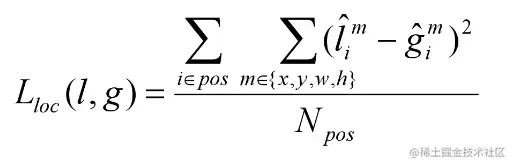L1、L2、Smooth L1 Regression as target detection Loss The shortcomings of ：

• The coordinates are calculated separately x、y、w、h The loss of , As a 4 Two different objects handle .bbox Of 4 This part should be discussed as a whole , But being treated independently .
• Sensitive to scale , The prediction frame and the real frame with different prediction effects may produce the same loss.

## 3. IOU Loss

### 3.1 IOU Loss principle

IOU Loss It's open mindedness in UnitBox A loss function calculation method of boundary box proposed in ,L1 、 L2 as well as Smooth L1 Loss Yes, it will bbox Four points to find loss Then I add , The correlation between coordinates is not considered . As shown in the figure below , The black box is the real box , The green box is the prediction box , Obviously, the prediction effect of the third box is better , But these three boxes have the same L2 loss, It's obviously unreasonable .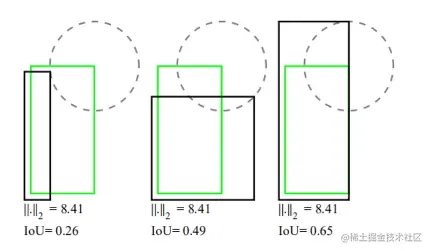IOU Loss take 4 Composed of points bbox Return as a whole , The design idea is as follows ：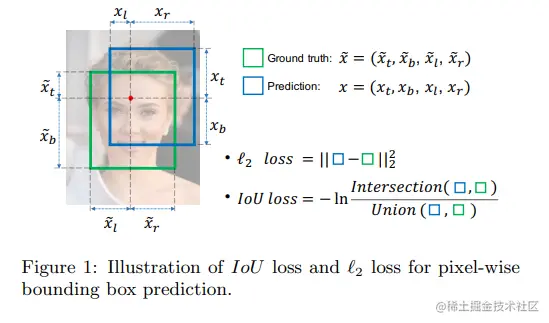The algorithm flow is as follows ：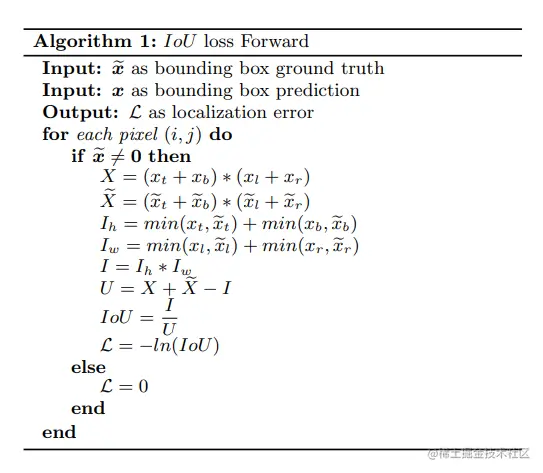For those prediction frames that are really real objects , Calculate the intersection area and combined area of the prediction frame and the real frame , Divide and take -log, Calculate to get IOU Loss. The higher the degree of coincidence between the prediction frame and the real frame ,loss The closer to 0, conversely loss The bigger it is , In this way Loss Function design is reasonable .

### 3.2 IOU Loss Code implementation

The code implementation is as follows ：

def iou_loss(pred, target, reduction='mean', eps=1e-6):
""" preds:[[x1,y1,x2,y2], [x1,y1,x2,y2],,,] bbox:[[x1,y1,x2,y2], [x1,y1,x2,y2],,,] reduction: "mean" or "sum" return: loss """
#  seek pred, target area
pred_widths = (pred[:, 2] - pred[:, 0] + 1.).clamp(0)
pred_heights = (pred[:, 3] - pred[:, 1] + 1.).clamp(0)
target_widths = (target[:, 2] - target[:, 0] + 1.).clamp(0)
target_heights = (target[:, 3] - target[:, 1] + 1.).clamp(0)
pred_areas = pred_widths * pred_heights
target_areas = target_widths * target_heights

#  seek pred, target Intersection area
inter_xmins = torch.maximum(pred[:, 0], target[:, 0])
inter_ymins = torch.maximum(pred[:, 1], target[:, 1])
inter_xmaxs = torch.minimum(pred[:, 2], target[:, 2])
inter_ymaxs = torch.minimum(pred[:, 3], target[:, 3])
inter_widths = torch.clamp(inter_xmaxs - inter_xmins + 1.0, min=0.)
inter_heights = torch.clamp(inter_ymaxs - inter_ymins + 1.0, min=0.)
inter_areas = inter_widths * inter_heights

#  seek iou
ious = torch.clamp(inter_areas / (pred_areas + target_areas - inter_areas), min=eps)
if reduction == 'mean':
loss = torch.mean(-torch.log(ious))
elif reduction == 'sum':
loss = torch.sum(-torch.log(ious))
else:
raise NotImplementedError

return loss
Copy code 

### 3.3 IOU Loss Analysis of advantages and disadvantages

• IOU Loss It can reflect the fitting effect of prediction frame and real frame .
• IOU Loss Scale invariance , Insensitive to scale .

shortcoming ：

• It is impossible to measure the loss caused by two completely disjoint boxes （iou Fixed for 0）.
• Two prediction frames with different shapes may produce the same loss（ same iou）.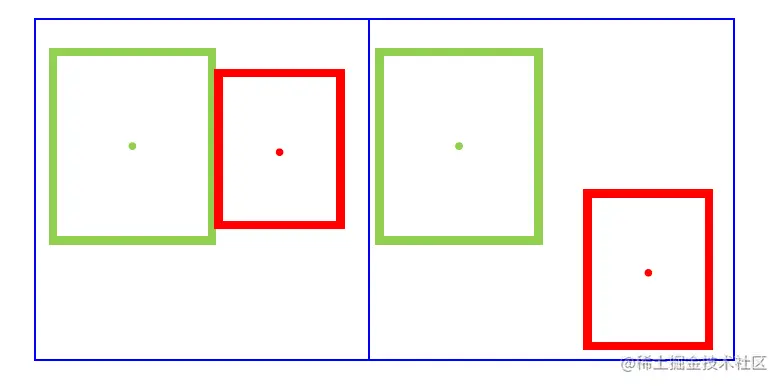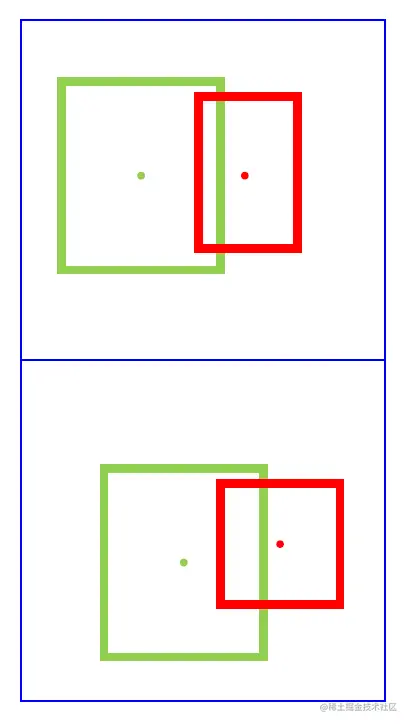## 4. GIOU Loss

### 4.1 GIOU Loss principle

GIOU The original intention of our design is to solve IOU Loss The problem is （ When the prediction frame does not intersect the real frame iou Constant is 0）, Designed a set of Generalized Intersection over Union Loss. stay IOU On the basis of ,GIOU You also need to find the smallest circumscribed rectangle of the prediction box and the real box , Then find the minimum circumscribed rectangle minus two prediction frames union The area of （ The area of the purple backslash area in the following figure ）, Definition GIOU by IOU The difference from the area just calculated .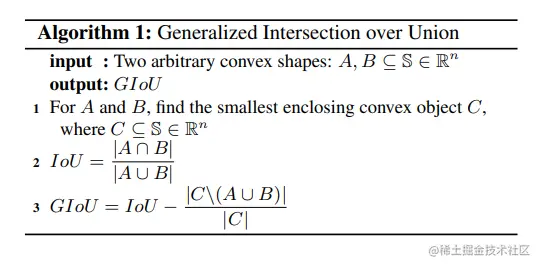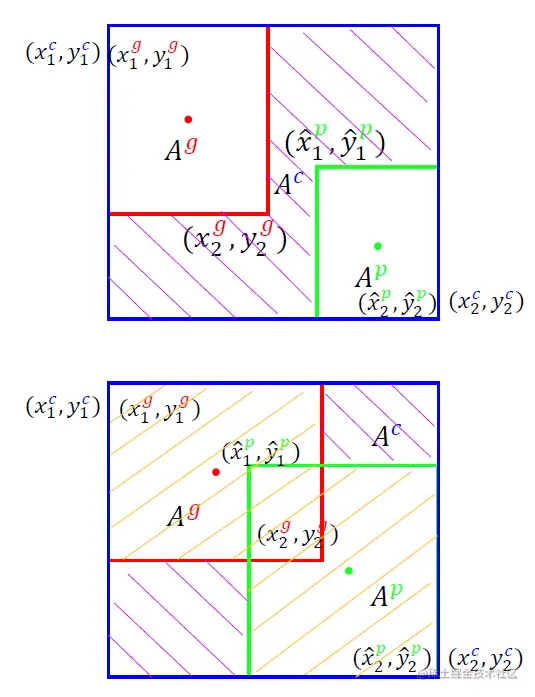Definition GIOU Loss = 1 - GIOU, be aware GIOU The scope is [-1, 1], that GIOU Loss The scope of [0, 2]. Whole GIOU Loss The algorithm flow is shown in the figure below ：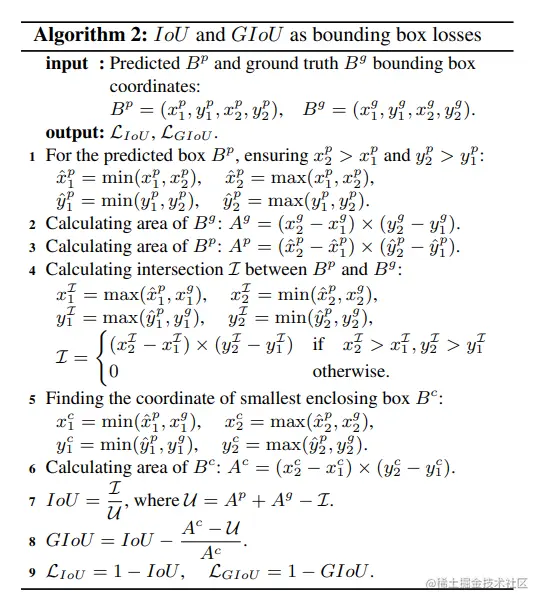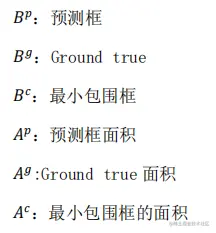### 4.2 GIOU Loss Code implementation

If you look a little confused , Just look at the code , The process is not complicated .

def giou_loss(pred, target, reduction='mean', eps=1e-6):
""" preds:[[x1,y1,x2,y2], [x1,y1,x2,y2],,,] bbox:[[x1,y1,x2,y2], [x1,y1,x2,y2],,,] reduction: "mean" or "sum" return: loss """
#  seek pred, target area
pred_widths = (pred[:, 2] - pred[:, 0] + 1.).clamp(0)
pred_heights = (pred[:, 3] - pred[:, 1] + 1.).clamp(0)
target_widths = (target[:, 2] - target[:, 0] + 1.).clamp(0)
target_heights = (target[:, 3] - target[:, 1] + 1.).clamp(0)
pred_areas = pred_widths * pred_heights
target_areas = target_widths * target_heights

#  seek pred, target Intersection area
inter_xmins = torch.maximum(pred[:, 0], target[:, 0])
inter_ymins = torch.maximum(pred[:, 1], target[:, 1])
inter_xmaxs = torch.minimum(pred[:, 2], target[:, 2])
inter_ymaxs = torch.minimum(pred[:, 3], target[:, 3])
inter_widths = torch.clamp(inter_xmaxs - inter_xmins + 1.0, min=0.)
inter_heights = torch.clamp(inter_ymaxs - inter_ymins + 1.0, min=0.)
inter_areas = inter_widths * inter_heights

#  seek iou
unions = pred_areas + target_areas - inter_areas
ious = torch.clamp(inter_areas / unions, min=eps)

#  Find the minimum circumscribed rectangle
outer_xmins = torch.minimum(pred[:, 0], target[:, 0])
outer_ymins = torch.minimum(pred[:, 1], target[:, 1])
outer_xmaxs = torch.maximum(pred[:, 2], target[:, 2])
outer_ymaxs = torch.maximum(pred[:, 3], target[:, 3])
outer_widths = (outer_xmaxs - outer_xmins + 1).clamp(0.)
outer_heights = (outer_ymaxs - outer_ymins + 1).clamp(0.)
outer_areas = outer_heights * outer_widths

gious = ious - (outer_areas - unions) / outer_areas
gious = gious.clamp(min=-1.0, max=1.0)
if reduction == 'mean':
loss = torch.mean(1 - gious)
elif reduction == 'sum':
loss = torch.sum(1 - gious)
else:
raise NotImplementedError
return loss
Copy code 

### 4.3 GIOU Loss Analysis of advantages and disadvantages

• GIOU Loss It's solved IOU Loss Problems in disjoint situations , In the target detection task, we can get higher accuracy .

shortcoming ：

• It is impossible to measure the box regression loss when there is an inclusion relationship , Here's the picture , The three regression boxes have the same GIOU Loss, But obviously the regression effect of the third box is better .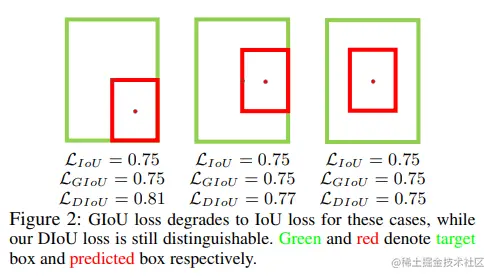## 5. DIOU Loss

### 5.1 DIOU Loss principle

In order to solve GIOU Loss There is no way to measure the of two boxes that fully contain the relationship loss The problem of ,DIOU Loss The distance between the centers of the two boxes is added to the loss function , use  The square of the distance between the center of the frame / The diagonal of the smallest outer rectangle （ The length of the red line / Blue line length ） To replace GIOU Loss Area ratio in .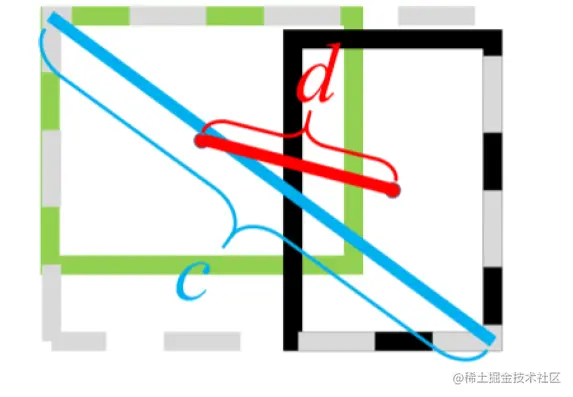DIOU Calculation formula ：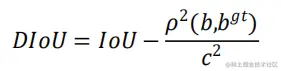DIOU Loss Calculation formula ：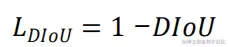### 5.2 DIOU Loss Code implementation

def diou_loss(pred, target, reduce='mean', eps=1e-6):
""" preds:[[x1,y1,x2,y2], [x1,y1,x2,y2],,,] bbox:[[x1,y1,x2,y2], [x1,y1,x2,y2],,,] reduction: "mean" or "sum" return: loss """
#  seek pred, target area
pred_widths = (pred[:, 2] - pred[:, 0] + 1.).clamp(0)
pred_heights = (pred[:, 3] - pred[:, 1] + 1.).clamp(0)
target_widths = (target[:, 2] - target[:, 0] + 1.).clamp(0)
target_heights = (target[:, 3] - target[:, 1] + 1.).clamp(0)
pred_areas = pred_widths * pred_heights
target_areas = target_widths * target_heights

#  seek pred, target Intersection area
inter_xmins = torch.maximum(pred[:, 0], target[:, 0])
inter_ymins = torch.maximum(pred[:, 1], target[:, 1])
inter_xmaxs = torch.minimum(pred[:, 2], target[:, 2])
inter_ymaxs = torch.minimum(pred[:, 3], target[:, 3])
inter_widths = torch.clamp(inter_xmaxs - inter_xmins + 1.0, min=0.)
inter_heights = torch.clamp(inter_ymaxs - inter_ymins + 1.0, min=0.)
inter_areas = inter_widths * inter_heights

#  seek iou
unions = pred_areas + target_areas - inter_areas + eps
ious = torch.clamp(inter_areas / unions, min=eps)

#  Find the minimum diagonal distance of circumscribed rectangle
outer_xmins = torch.minimum(pred[:, 0], target[:, 0])
outer_ymins = torch.minimum(pred[:, 1], target[:, 1])
outer_xmaxs = torch.maximum(pred[:, 2], target[:, 2])
outer_ymaxs = torch.maximum(pred[:, 3], target[:, 3])
outer_diag = torch.clamp((outer_xmaxs - outer_xmins + 1.), min=0.) ** 2 + \
torch.clamp((outer_ymaxs - outer_ymins + 1.), min=0.) ** 2 + eps

#  seek pred And target The center distance of the frame
c_pred = ((pred[:, 0] + pred[:, 2]) / 2, (pred[:, 1] + pred[:, 3]) / 2)
c_target = ((target[:, 0] + target[:, 2]) / 2, (target[:, 1] + target[:, 3]) / 2)
distance = (c_pred - c_target + 1.) ** 2 + (c_pred - c_target + 1.) ** 2

#  seek diou loss
dious = ious - distance / outer_diag
if reduce == 'mean':
loss = torch.mean(1 - dious)
elif reduce == 'sum':
loss = torch.sum(1 - dious)
else:
raise NotImplementedError

return loss
Copy code 

### 5.3 DIOU Loss Analysis of advantages and disadvantages

• DIOU Loss It's solved GIOU Loss The problem that loss cannot be measured in the case of full inclusion relationship , In the target detection task, we can further get higher accuracy .

shortcoming ：

• It is impossible to measure that the center point of two boxes containing the relationship is close to 、 Losses of the same area but different shapes , Here's the picture , The center points of the two boxes coincide , The left and right prediction red boxes have the same area but different shapes , both DIOU Loss identical , But obviously the latter fits better .

## 6. CIOU Loss

### 6.1 CIOU Loss principle

CIOU Loss and DIOU Loss It was put forward in the same article , stay DIOU Loss On the basis of ,CIOU Loss The shape of prediction box is considered （ Aspect ratio ） Whether it is consistent with the real box , It's right DIOU Loss Very good supplement .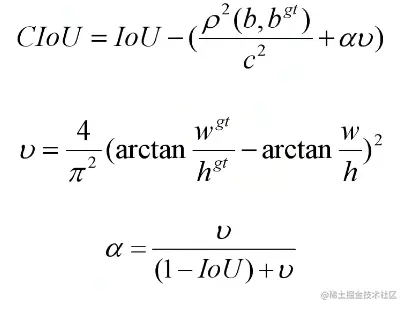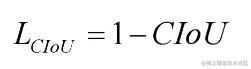Notice the new αv in ,IOU The bigger it is , The smaller the denominator ,α The bigger it is , That is, the higher the specific gravity of the aspect ratio . In this way , Put the overlapping area 、 The center distance and frame shape are integrated into one loss In the function .

### 6.2 CIOU Loss Code implementation

def ciou_loss(pred, target, reduce='mean', eps=1e-6):
""" preds:[[x1,y1,x2,y2], [x1,y1,x2,y2],,,] bbox:[[x1,y1,x2,y2], [x1,y1,x2,y2],,,] reduction: "mean" or "sum" return: loss """
#  seek pred, target area
pred_widths = (pred[:, 2] - pred[:, 0] + 1.).clamp(0)
pred_heights = (pred[:, 3] - pred[:, 1] + 1.).clamp(0)
target_widths = (target[:, 2] - target[:, 0] + 1.).clamp(0)
target_heights = (target[:, 3] - target[:, 1] + 1.).clamp(0)
pred_areas = pred_widths * pred_heights
target_areas = target_widths * target_heights

#  seek pred, target Intersection area
inter_xmins = torch.maximum(pred[:, 0], target[:, 0])
inter_ymins = torch.maximum(pred[:, 1], target[:, 1])
inter_xmaxs = torch.minimum(pred[:, 2], target[:, 2])
inter_ymaxs = torch.minimum(pred[:, 3], target[:, 3])
inter_widths = torch.clamp(inter_xmaxs - inter_xmins + 1.0, min=0.)
inter_heights = torch.clamp(inter_ymaxs - inter_ymins + 1.0, min=0.)
inter_areas = inter_widths * inter_heights

#  seek iou
unions = pred_areas + target_areas - inter_areas + eps
ious = torch.clamp(inter_areas / unions, min=eps)

#  Find the minimum diagonal distance of circumscribed rectangle
outer_xmins = torch.minimum(pred[:, 0], target[:, 0])
outer_ymins = torch.minimum(pred[:, 1], target[:, 1])
outer_xmaxs = torch.maximum(pred[:, 2], target[:, 2])
outer_ymaxs = torch.maximum(pred[:, 3], target[:, 3])
outer_diag = torch.clamp((outer_xmaxs - outer_xmins + 1.), min=0.) ** 2 + \
torch.clamp((outer_ymaxs - outer_ymins + 1.), min=0.) ** 2 + eps

#  seek pred And target The center distance of the frame
c_pred = ((pred[:, 0] + pred[:, 2]) / 2, (pred[:, 1] + pred[:, 3]) / 2)
c_target = ((target[:, 0] + target[:, 2]) / 2, (target[:, 1] + target[:, 3]) / 2)
distance = (c_pred - c_target + 1.) ** 2 + (c_pred - c_target + 1.) ** 2

#  Find the loss on the shape of the prediction box
w_pred, h_pred = pred[:, 2] - pred[:, 0], pred[:, 3] - pred[:, 1] + eps
w_target, h_target = target[:, 2] - target[:, 0], target[:, 3] - target[:, 1] + eps
factor = 4 / (math.pi ** 2)
v = factor * torch.pow(torch.atan(w_pred / h_pred) - torch.atan(w_target / h_target), 2)
alpha = v / (1 - ious + v)

#  seek ciou loss
cious = ious - distance / outer_diag - alpha * v
if reduce == 'mean':
loss = torch.mean(1 - cious)
elif reduce == 'sum':
loss = torch.sum(1 - cious)
else:
raise NotImplementedError

return loss
Copy code 

## 7. Summary and effect of regression loss function of target detection frame

An excellent positioning loss should be considered as follows 3 One factor ：

• Overlap area
• Center distance
• Aspect ratio

As shown in the figure below , The loss function is in YOLOv3 Performance effect on , Can be observed IOU Loss、GIOU Loss、DIOU Loss and CIOU Loss In turn, it has a certain accuracy improvement effect ：